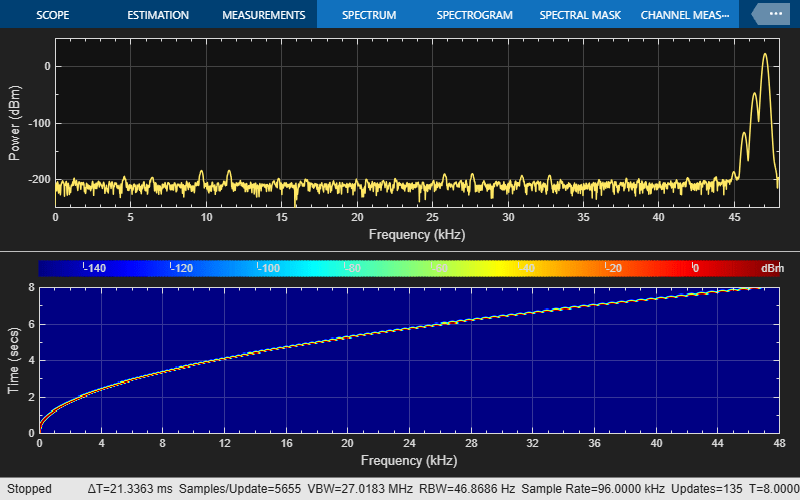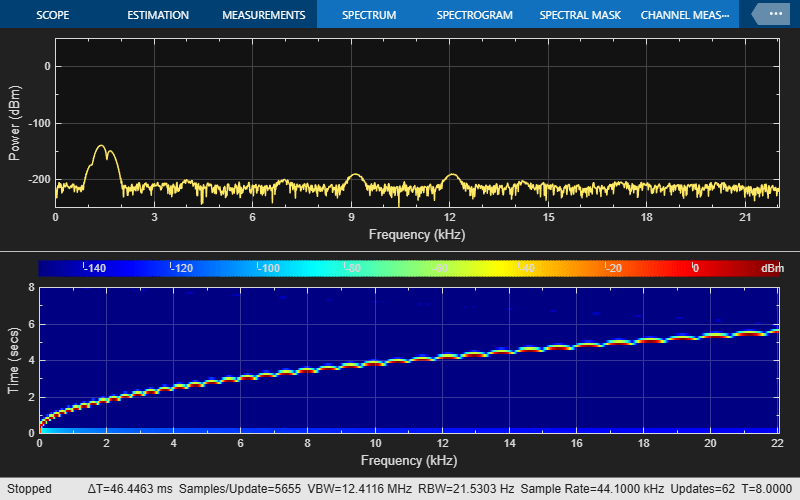# Multistage Sample-Rate Conversion of Audio Signals

This example shows how to use a multistage/multirate approach to sample rate conversion between different audio sampling rates.

The example uses `dsp.SampleRateConverter`. This component automatically determines how many stages to use and designs the filter required for each stage in order to perform the sample rate conversion in a computationally efficient manner.

This example focuses on converting an audio signal sampled at 96 kHz (DVD quality) to an audio signal sampled at 44.1 kHz (CD quality).The comparison is done using data sampled at 96 kHz available online at http://src.infinitewave.ca/. In this example, the 96 kHz chirp signal is generated locally so that no download is needed.

### Setup

Define some parameters to be used throughout the example.

```frameSize = 64; inFs = 96e3; ```

### Reading the 96 kHz File

The website above has 3 sets of files at different qualities in order to perform the comparison. In this example the focus will be on one of the files only: `Swept_int.wav`. This file contains a chirp sine wave sweeping from 0 Hz to 48 kHz over the course of 8 seconds. The format of the file is 32-bit integers, given it a very high dynamic range.

Here you create a System object to read from the audio file and determine the file's audio sampling rate. If you want to use the wav file instead of `dsp.Chirp`, uncomment the lines below and skip the call to `dsp.Chirp`.

source = dsp.AudioFileReader('Swept_int.wav', ... 'SamplesPerFrame',frameSize, ... 'OutputDataType','double');

### Generating the 96 kHz Signal

In lieu of downloading the `Swept_int.wav` file, you can also generate the chirp signal using `dsp.Chirp` as follows:

```source = dsp.Chirp('InitialFrequency',0,'TargetFrequency',48e3, ... 'SweepTime',8,'TargetTime',8,'SampleRate',inFs, ... 'SamplesPerFrame',frameSize,'Type','Quadratic'); ```

### Create Spectrum Analyzers

Create two spectrum analyzers. These will be used to visualize the frequency content of the original signal as well as that of the signals converted to 44.1 kHz.

```SpectrumAnalyzer44p1 = dsp.SpectrumAnalyzer( ... 'SampleRate',44100, ... 'ViewType','Spectrum and spectrogram', ... 'TimeSpanSource','Property','TimeSpan',8, ... 'Window','Kaiser','SidelobeAttenuation',220, ... 'YLimits',[-250, 50],'ColorLimits',[-150, 20], ... 'PlotAsTwoSidedSpectrum',false); SpectrumAnalyzer96 = dsp.SpectrumAnalyzer( ... 'SampleRate',96000, ... 'ViewType','Spectrum and spectrogram', ... 'TimeSpanSource','Property','TimeSpan',8, ... 'Window','Kaiser','SidelobeAttenuation',220, ... 'YLimits',[-250, 50],'ColorLimits',[-150, 20], ... 'PlotAsTwoSidedSpectrum',false); ```

### Spectrum of Original Signal Sampled at 96 kHz

The loop below plots the spectrogram and power spectrum of the original 96 kHz signal. The chirp signal starts at 0 and sweeps to 48 kHz over a simulated time of 8 seconds.

```NFrames = 8*inFs/frameSize; for k = 1:NFrames sig96 = source(); % Source SpectrumAnalyzer96(sig96); % Spectrogram end release(source) release(SpectrumAnalyzer96) ```### Setting up the Sample Rate Converter

In order to convert the signal, `dsp.SampleRateConverter` is used. A first attempt sets the bandwidth of interest to 40 kHz, i.e. to cover the range [-20 kHz, 20 kHz]. This is the usually accepted range that is audible to humans. The stopband attenuation for the filters to be used to remove spectral replicas and aliased replicas is left at the default value of 80 dB.

```BW40 = 40e3; OutFs = 44.1e3; SRC40kHz80dB = dsp.SampleRateConverter('Bandwidth',BW40, ... 'InputSampleRate',inFs,'OutputSampleRate',OutFs); ```

### Analysis of the Filters Involved in the Conversion

Use `info` to get information on the filters that are designed to perform the conversion. This reveals that the conversion will be performed in two steps. The first step involves a decimation by two filter which converts the signal from 96 kHz to 48 kHz. The second step involves an FIR rate converter that interpolates by 147 and decimates by 160. This results in the 44.1 kHz required. The `freqz` command can be used to visualize the combined frequency response of the two stages involved. Zooming in reveals that the passband extends up to 20 kHz as specified and that the passband ripple is in the milli-dB range (less than 0.003 dB).

```info(SRC40kHz80dB) [H80dB,f] = freqz(SRC40kHz80dB,0:10:25e3); plot(f,20*log10(abs(H80dB)/norm(H80dB,inf))) xlabel('Frequency (Hz)') ylabel('Magnitude (dB)') axis([0 25e3 -140 5]) ```
```ans = 'Overall Interpolation Factor : 147 Overall Decimation Factor : 320 Number of Filters : 2 Multiplications per Input Sample: 42.334375 Number of Coefficients : 8618 Filters: Filter 1: dsp.FIRDecimator - Decimation Factor : 2 Filter 2: dsp.FIRRateConverter - Interpolation Factor: 147 - Decimation Factor : 160 ' ```### Asynchronous Buffer

The sample rate conversion from 96 kHz to 44.1 kHz produces 147 samples for every 320 input samples. Because the chirp signal is generated with frames of 64 samples, an asynchronous buffer is needed. The chirp signal is written 64 samples at a time, and whenever there are enough samples buffered, 320 of them are read and fed to the sample rate converter.

```buff = dsp.AsyncBuffer; ```

### Main Processing Loop

The loop below performs the sample rate conversion in streaming fashion. The computation is fast enough to operate in real time if need be.

The spectrogram and power spectrum of the converted signal are plotted. The extra lines in the spectrogram correspond to spectral aliases/images remaining after filtering. The replicas are attenuated by better than 80 dB as can be verified with the power spectrum plot.

```srcFrameSize = 320; for k = 1:NFrames sig96 = source(); % Generate chirp write(buff,sig96); % Buffer data if buff.NumUnreadSamples >= srcFrameSize sig96buffered = read(buff,srcFrameSize); sig44p1 = SRC40kHz80dB(sig96buffered); % Convert sample-rate SpectrumAnalyzer44p1(sig44p1); % View spectrum of converted signal end end release(source) release(SpectrumAnalyzer44p1) release(buff) ```### A More Precise Sample Rate Converter

In order to improve the sample rate converter quality, two changes can be made. First, the bandwidth can be extended from 40 kHz to 43.5 kHz. This in turn requires filters with a sharper transition. Second, the stopband attenuation can be increased from 80 dB to 160 dB. Both these changes come at the expense of more filter coefficients over all as well as more multiplications per input sample.

```BW43p5 = 43.5e3; SRC43p5kHz160dB = dsp.SampleRateConverter('Bandwidth',BW43p5, ... 'InputSampleRate',inFs,'OutputSampleRate',OutFs, ... 'StopbandAttenuation',160); ```

### Analysis of the Filters Involved in the Conversion

The previous sample rate converter involved 8618 filter coefficients and a computational cost of 42.3 multiplications per input sample. By increasing the bandwidth and stopband attenuation, the cost increases substantially to 123896 filter coefficients and 440.34 multiplications per input sample. The frequency response reveals a much sharper filter transition as well as larger stopband attenuation. Moreover, the passband ripple is now in the micro-dB scale. NOTE: this implementation involves the design of very long filters which takes several minutes to complete. However, this is a one time cost which happens offline (before the actual sample rate conversion).

```info(SRC43p5kHz160dB) [H160dB,f] = freqz(SRC43p5kHz160dB,0:10:25e3); plot(f,20*log10(abs(H160dB)/norm(H160dB,inf))); xlabel('Frequency (Hz)') ylabel('Magnitude (dB)') axis([0 25e3 -250 5]) ```
```ans = 'Overall Interpolation Factor : 147 Overall Decimation Factor : 320 Number of Filters : 2 Multiplications per Input Sample: 440.340625 Number of Coefficients : 123896 Filters: Filter 1: dsp.FIRDecimator - Decimation Factor : 2 Filter 2: dsp.FIRRateConverter - Interpolation Factor: 147 - Decimation Factor : 160 ' ```### Main Processing Loop

The processing is repeated with the more precise sample rate converter.

Once again the spectrogram and power spectrum of the converted signal are plotted. Notice that the imaging/aliasing is attenuated enough that they are not visible in the spectrogram. The power spectrum shows spectral aliases attenuated by more than 160 dB (the peak is at about 20 dB).

```for k = 1:NFrames sig96 = source(); % Generate chirp over = write(buff,sig96); % Buffer data if buff.NumUnreadSamples >= srcFrameSize [sig96buffered,under] = read(buff,srcFrameSize); sig44p1 = SRC43p5kHz160dB(sig96buffered); % Convert sample-rate SpectrumAnalyzer44p1(sig44p1); % View spectrum of converted signal end end release(source) release(SpectrumAnalyzer44p1) release(buff) ```## SupportGet trial now